# Orange colour

In order for the painter to get orange, he has to mix yellow and red in a ratio of 3: 4. How many liters of orange did he mix if he used 8 liters of red?

x =  14 l

### Step-by-step explanation: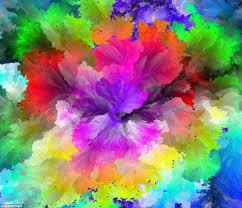Did you find an error or inaccuracy? Feel free to write us. Thank you!Tips to related online calculators
Check out our ratio calculator.
Do you have a linear equation or system of equations and looking for its solution? Or do you have a quadratic equation?
Tip: Our volume units converter will help you with the conversion of volume units.

## Related math problems and questions:

• The painterIn order for the painter to get the desired color, he must mix green and yellow in a ratio of 4: 7. If it has 28 l of green color, how many liters of yellow color should he add? How many liters of mixed color does he get?
• Cuboid - ratiosThe sizes of the edges of the cuboid are in the ratio 2: 3: 5. The smallest wall has an area of 54 cm2. Calculate the surface area and volume of this cuboid.
• PeppersIn the box are yellow (a), green (b), and red (c) peppers. Their amount is in a ratio of 2:4:1. Most are yellow peppers and green the least. Calculate the number of peppers each type if the total number of peppers is 70.
• Two masters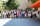The two masters will make as many parts as five apprentices at the same time. An eight-hour shift begins at 6 o'clock. When can a master finish the job to produce just as much as an apprentice for the whole shift?
• Sick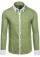Six seamstresses should make 60 shirts in five business days. After three days two seamstresses were sick. How many days have the remaining seamstresses to finish contract?
• Painter Pavel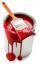Painter Pavel painted the fence for 16 hours and painter Petr the same fence painted for 13 h. How long should take painted the fence together?
• Ratio of edgesThe dimensions of the cuboid are in a ratio 3: 1: 2. The body diagonal has a length of 28 cm. Find the volume of a cuboid.
• Eight blocks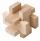Dana had the task to save the eight blocks of these rules: 1. Between two red cubes must be a different color. 2. Between two blue must be two different colors. 3. Between two green must be three different colors. 4. Between two yellow blocks must be four
• Markus painterMarkus used ¾ liter of paint to cover 10 ½ square meters of wall. How many liters of paint is needed to cover 12 ¼ square meters of wall?
• The sidesThe sides of the rectangle are in a ratio of 3: 5 and its circumference measures 72 cm. Calculate: a) the size of both sides of the rectangle b) the area of the rectangle c) the length of the diagonals
• SolutionWhat amount of 80% solution and what amount of water is needed to mix in order to obtain two liters of 60% solution of this mixture?
• Trio ratioHans, Alena, and Thomas have a total of 740 USD. Hans and Alena split in the ratio 5: 6 and Alena and Thomas in the ratio 4: 5. How much will everyone get?
• ISO triangleCalculate the area of an isosceles triangle KLM if its sides' length is in the ratio k:l:m = 4:4:3 and has perimeter 377 mm.
• Isosceles trapezoidCalculate the content of an isosceles trapezoid whose bases are at ratio 5:3, the arm is 6cm long and it is 4cm high.
• Sides ratioCalculate the circumference of a triangle with area 84 cm2 if a:b:c = 10:17:21
• Two diggersTwo diggers should dig a ditch. If each of them worked just one-third of the time that the other digger needs, they'd dig up a 13/18 ditch together. Find the ratio of the performance of these two diggers.
• TrapezoidArea of trapezoid is 135 cm2. Sides a, c and height h are in a ratio 6:4:3. How long are a,c and h? Make calculation...